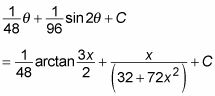##### Calculus II For DummiesWhen the function you’re integrating includes a term of the form (a2 + x2)n, draw your trigonometry substitution triangle for the tangent case. For example, suppose that you want to evaluate the following integral: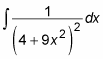This is a tangent case, because a constant plus a multiple of x2 is being raised to a power (–2). Here’s how you use trig substitution to integrate:

1. Draw the trig substitution triangle for the tangent case.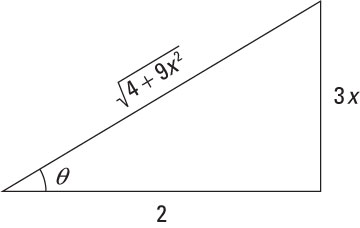The figure shows you how to fill in the triangle for the tangent case. Notice that the radical of what’s inside the parentheses goes on the hypotenuse of the triangle. Then, to fill in the other two sides of the triangle, use the square roots of the two terms inside the radical — that is, 2 and 3x. Place the constant term 2 on the adjacent side and the variable term 3x on the opposite side.

With the tangent case, make sure not to mix up your placement of the variable and the constant.

2. Identify the separate pieces of the integral (including dx) that you need to express in terms of theta.

In this case, the function contains two separate pieces that contain x: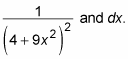3. Express these pieces in terms of trig functions of theta.

In the tangent case, all trig functions should be initially expressed as tangents and secants.

To represent the rational portion as a trig function of theta, build a fraction using the radical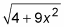as the numerator and the constant 2 as the denominator. Then set this fraction equal to the appropriate trig function: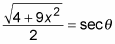Because this fraction is the hypotenuse of the triangle over the adjacent side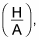it’s equal to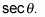Now use algebra and trig identities to tweak this equation into shape: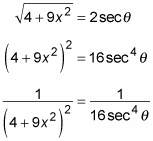Next, express dx as a trig function of theta. To do so, build another fraction with the variable 3x in the numerator and the constant 2 in the denominator: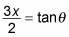This time, the fraction is the opposite side of the triangle over the adjacent side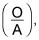so it equals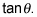Now solve for x and then differentiate: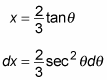4. Express the integral in terms of theta and evaluate it: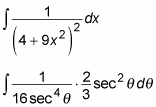Now some cancellation and reorganization turns this nasty-looking integral into something manageable: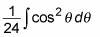At this point, you can evaluate this integral: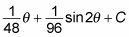So here’s the substitution: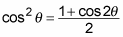And here is the antiderivative: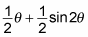5. Change the two theta terms back into x terms:

You need to find a way to express theta in terms of x. Here’s the simplest way: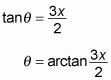So here’s a substitution that gives you an answer: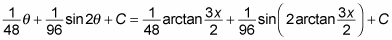This answer is valid, but most professors won’t be crazy about that ugly second term, with the sine of an arctangent. To simplify it, apply the double-angle sine formula to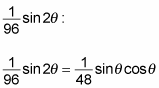Now use your trig substitution triangle to substitute values for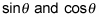in terms of x: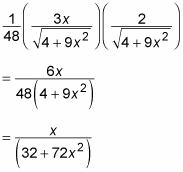Finally, use this result to express the answer in terms of x: